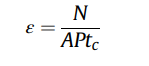# How calculate net area in root

Hello , please can anyone explain me how calculate net area in root ?

Net area ? what do you mean ?

i need to calculate detector efficiency and the expression of this iswith N IS is the net area at the interest peak energy

I am not sure there is a predefined function in ROOT to compute such formulae.
May be @moneta has an idea about it.

1 Like

I suppose that’s the integral? You can use `TF1::Integral()` if it’s coming from a fit. We’ll need a bit more context to be able to help, as is I might just reply "You want to divide N by A times Ptc? Use `/` and `*`"